## Integrating Factors

If a differential equation of the form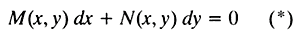is not exact as written, then there exists a function μ( x,y) such that the equivalent equation obtained by multiplying both sides of (*) by μ,is exact. Such a function μ is called an integrating factor of the original equation and is guaranteed to exist if the given differential equation actually has a solution. Integrating factors turn nonexact equations into exact ones. The question is, how do you find an integrating factor? Two special cases will be considered.

• Case 1:

Consider the differential equation M dx + N dy = 0. If this equation is not exact, then M y will not equal N x ; that is, M y N x ≠ 0. However, if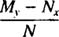is a function of x only, let it be denoted by ξ( x). Thenwill be an integrating factor of the given differential equation.

• Case 2:

Consider the differential equation M dx + N dy = 0. If this equation is not exact, then M y will not equal N x ; that is, M yN x ≠ 0. = 0. However, if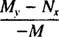is a function of y only, let it be denoted by ψ( y). Thenwill be an integrating factor of the given differential equation.

Example 1: The equation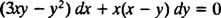is not exact, since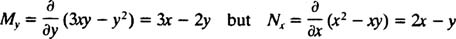However, note thatis a function of x alone. Therefore, by Case 1,will be an integrating factor of the differential equation. Multiplying both sides of the given equation by μ = x yieldswhich is exact becauseSolving this equivalent exact equation by the method described in the previous section, M is integrated with respect to x,and N integrated with respect to y:(with each “constant” of integration ignored, as usual). These calculations clearly giveas the general solution of the differential equation.

Example 2: The equation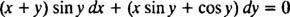is not exact, since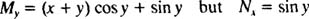However, note that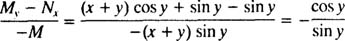is a function of y alone (Case 2). Denote this function by ψ( y); sincethe given differential equation will haveas an integrating factor. Multiplying the differential equation through by μ = (sin y) −1 yieldswhich is exact because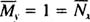To solve this exact equation, integrate M with respect to x and integrate N with respect to y, ignoring the “constant” of integration in each case:These integrations imply thatis the general solution of the differential equation.

Example 3: Solve the IVPThe given differential equation is not exact, sinceHowever, note thatwhich can be interpreted to be, say, a function of x only; that is, this last equation can be written as ξ( x) ≡ 2. Case 1 then says that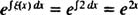will be an integrating factor. Multiplying both sides of the differential equation by μ( x) = e 2 x yieldswhich is exact because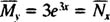Now, sinceand(with the “constant” of integration suppressed in each calculation), the general solution of the differential equation is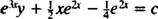The value of the constant c is now determined by applying the initial condition y(0) = 1: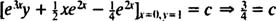Thus, the particular solution is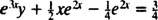which can be expressed explicitly as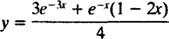Example 4: Given that the nonexact differential equation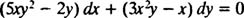has an integrating factor of the form μ( x,y) = x a y b for some positive integers a and b, find the general solution of the equation.

Since there exist positive integers a and b such that x a y b is an integrating factor, multiplying the differential equation through by this expression must yield an exact equation. That is,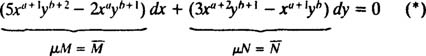is exact for some a and b. Exactness of this equation meansBy equating like terms in this last equation, it must be the case that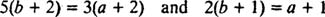The simultaneous solution of these equations is a = 3 and b = 1.

Thus the integrating factor x a y b is x 3 y, and the exact equation M dx + N dy = 0 readsNow, sinceand(ignoring the “constant” of integration in each case), the general solution of the differential equation (*)—and hence the original differential equation—is clearly